# Designs with multiple regressors and contrasts

### Dependencies and setup

This walkthrough uses CANlab object-oriented analysis functions with SPM12 basic functions (e.g., image reading/writing, HRF) in Matlab.
Do you have the relevant tools on your Matlab path?
which create_design_single_event
/Users/torwager/Documents/GitHub/CanlabCore/CanlabCore/Model_building_tools/create_design_single_event.m
If you get an error, you don't! You need the Canlab Core repository. See this page for setup instructions.
You also need the Statistics and Machine Learning Toolbox in Matlab. Try this to check:
if isempty(ver('stats')), warning('You do not have the statistics toolbox on your Matlab path. You may/will get errors'); end

### Experimental design

In the last lab, we constructed a single-subject fMRI design for a single regressor, responses to famous faces, in a sparse event-related design (3 sec face presentation followed by 18 sec inter-stimulus interval rest).
We constructed a model (X), which is the predicted fMRI signal in a brain area that responds to faces, and fit it to the data. Fitting the model involves solving the equation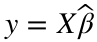, where y is a brain time series (a vector), Xis the model or "design matrix", and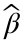is the unknown vector of regression slopes or "parameters".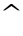or "hat" means something that is estimated from the data during model fitting. This model is fit to a single brain time series from a single person - for example, the average signal in a person's pre-identified "fusiform face area":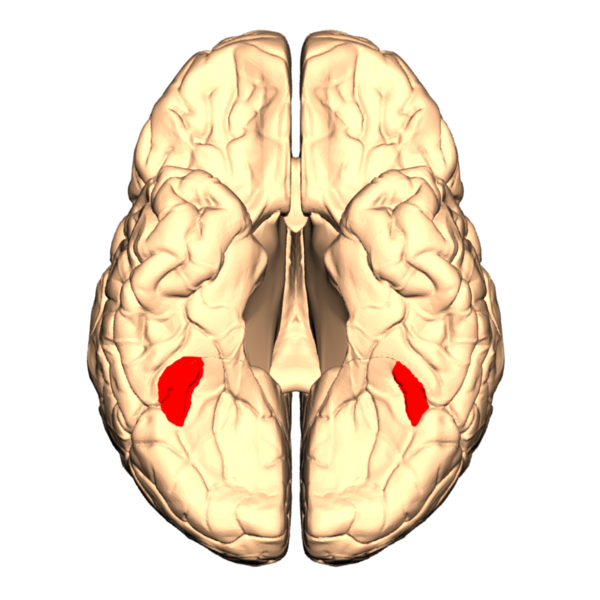Now, we'll extend this to designs with multiple conditions. Let's say that we're interested in comparing upright and inverted faces: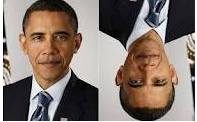### Create a two-event design

We'll create a random event-related design. The CANlab function create_random_er_design gives us lots of flexibility to create designs with different parameters and options.
In the code below, one event will be presented each ISI sec, for eventduration sec.
The variable freqConditions controls how many conditions there are, and what their relative frequencies are. They are entered in proportions (0 to 1) and will often sum to 1 (for 100% of the trials). If they don't, the remainder (0.2 in this case, as 0.4 + 0.4 sums to 0.8) will be assigned to rest - that is, no event type. This effectively randomizes the time between event presentations (in units of ISI sec, or 4 sec here). This randomization is often called "jitter" in the fMRI literature.
TR = 1; % Repetition time for scans; one image every TR; in seconds
ISI = 4; % Inter-stimulus interval in sec (min time between events)
eventduration = 3; % Duration of 'neural' events in sec
scanLength = 360; % Scan length in sec
HPlength = 128; % High-pass filter length in sec
dononlin = 0; % Nonlinear saturation model (0 = no, 1 = yes)
freqConditions = [.4 .4]; % Frequencies of each condition, from 0 - 1
HPlength = 128;
dononlin = 0;
create_figure('design');
[X, e, ons] = create_random_er_design(TR, ISI, eventduration, freqConditions, HPlength, dononlin, 'scanLength', scanLength);
axis tight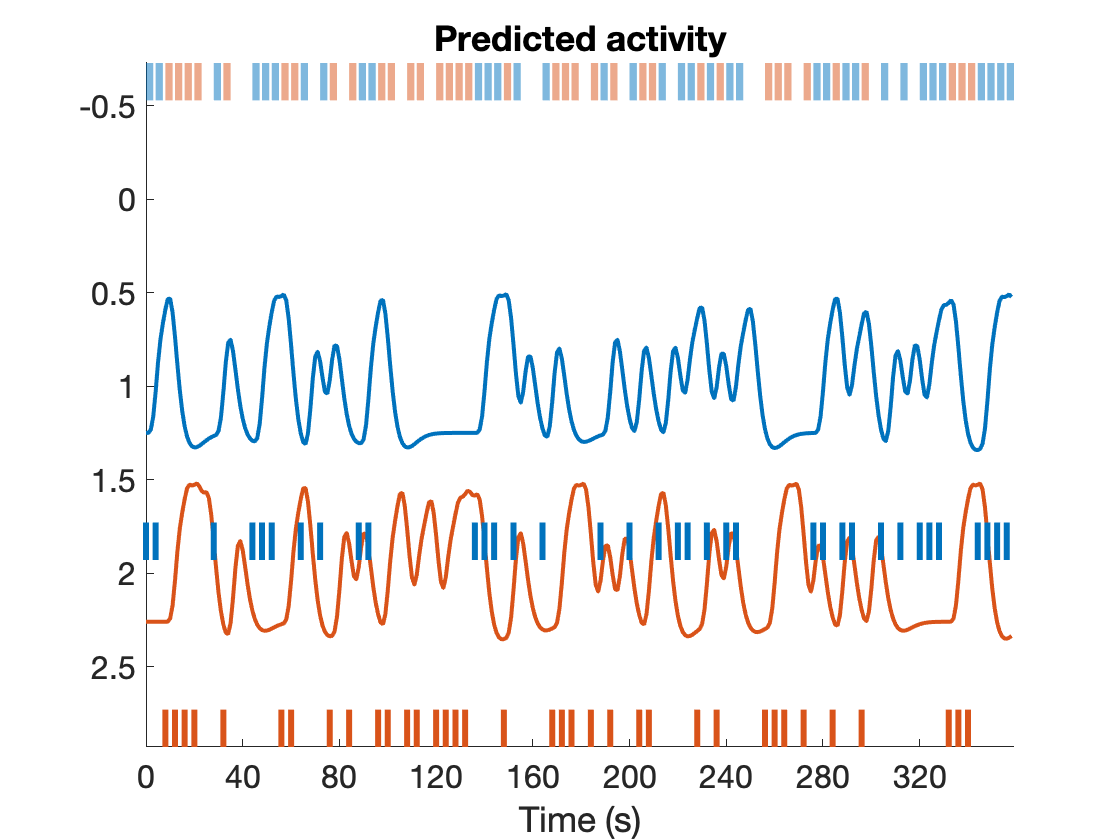disp(['e = ' num2str(e)])
e = 621.1374
As before, X is the design matrix. Which is the upright face and which is inverted are arbitrary here. Let's assign "upright" to column 1, and "inverted" to column 2.
We now have another output variable, ons, which contains a cell array of event onsets and durations (in seconds) for each event. ons{1} corresponds to Condition 1, ons{2} to Condition 2, and so forth. ons{i} can be an [n x 2] array, where the first column is onset time for each event, and the second column is the event duration in seconds. The onsets can be useful for plugging into fMRI analysis software (like SPM) and saving for descriptive purposes and recreating designs later. By convention, time 0 marks the start of the first fMRI image acquisition.
The table is a useful type of object for storing many kinds of data. Let's create and display a table of the onsets here.
% Create a table object (t) with event onsets and display it
t = table(ons{1}(:, 1), ons{1}(:, 2), ons{2}(:, 1), ons{2}(:, 2), 'VariableNames', {'Evt1_Time' 'Evt1_Dur' 'Evt2_Time' 'Evt2_Dur'});
disp(t);
Evt1_Time Evt1_Dur Evt2_Time Evt2_Dur _________ ________ _________ ________ 0 3 8 3 4 3 12 3 28 3 16 3 44 3 20 3 48 3 32 3 52 3 56 3 64 3 60 3 72 3 76 3 88 3 84 3 92 3 96 3 136 3 100 3 140 3 108 3 144 3 112 3 152 3 120 3 164 3 124 3 188 3 128 3 200 3 132 3 212 3 148 3 220 3 168 3 224 3 172 3 232 3 176 3 240 3 184 3 244 3 192 3 276 3 204 3 280 3 208 3 288 3 228 3 292 3 236 3 304 3 256 3 312 3 260 3 320 3 264 3 324 3 272 3 328 3 284 3 344 3 296 3 348 3 332 3 352 3 336 3 356 3 340 3
e is the "efficiency", a relative metric of how good the design is compared with others with the same number of time points. We can use efficiency to explore which choices make a "better" design and which create problems. We'll explore this a bit later.

1. Create a heat map of X, as in the previous lab. What does each column represent (i.e., a task event type, etc.)?
2. How many elements will thevector have in the regression? Which one corresponds to the intercept?

### More event types: Factorial design example

Changing freqConditions controls the number of event types (conditions) and how dense they are, or how many of them occur in a given time frame.
Let's say we want a factorial design with 4 event types: [Famous vs. Non-famous] x [Upright vs. Inverted]. There are two factors here, with two levels (unique values) for each factor.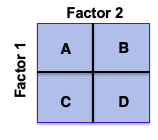There are four cells in this design, one for each unique combination of levels across the two factors. The strategy is to model each cell as a unique event type (condition), and use contrasts to estimate the main effects of each factor and their interaction. We'll deal with contrasts below.
Here, A and B might be "famous upright" and "famous inverted", respectively, and C and D "non-famous upright" and "non-famous inverted". These assignments are not set in stone (i can choose to assign "famous upright" and "famous inverted" to C and D, or to A and C, for example) -- but I should use a consistent, conceptual mapping - so we'll go with this.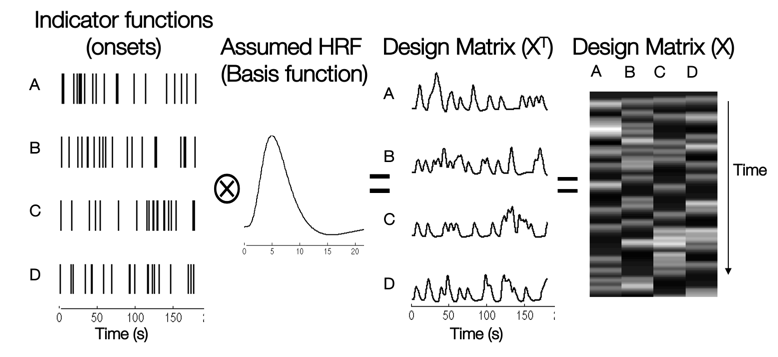With more event types, we have to start to worry about several things: (1) Do we have enough events of each type, and more precisely enough predictor variance, to detect effects? (2) Are the predictors colinear, in the sense that some predictors are redundant with others?

1. Try changing the design to have four event types. Copy/paste the relevant code from above here, so you can generate a new design plot.
freqConditions = [.2 .2 .2 .2]; % Frequencies of each condition, from 0 - 1
create_figure('design');
[X, e, ons] = create_random_er_design(TR, ISI, eventduration, freqConditions, HPlength, dononlin, 'scanLength', scanLength);
axis tight
2. How correlated are the regressors? hint: corr in Matlab. Make a plot of the correlation matrix (hint: imagesc and colorbar are useful functions here)

### Contrasts

Contrasts are linear combinations across the parameter estimates,. We can specify those with a matrix C, which contains one or more columns. Each column is a different contrast. The rules for contrasts are explained in this video lecture.
For example, this matrix will create a set of ANOVA contrasts for main effects and their interaction: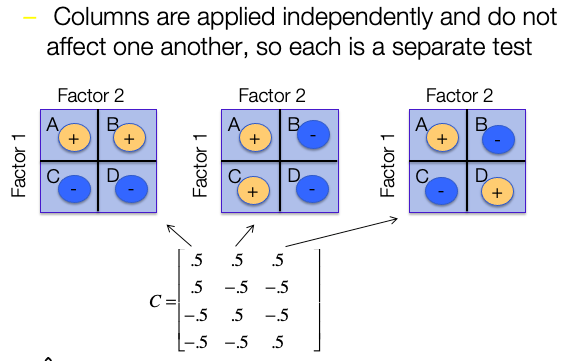C = [.5 .5 -.5 -.5 0]'; % one contrast. The ' means "transpose", so C becomes a column vector
C = [.5 .5 -.5 -.5 0; .5 -.5 .5 -.5 0; .5 -.5 -.5 .5 0]'; % ANOVA contrasts
Here, I've added an extra 0 to the end. Why? We need to add a weight for the intercept too! Because we don't care about averaging some fraction of the intercept in with the rest of the signal, we'll use 0 so that its value will not influence the contrast values.
In practice, we'll apply the contrasts to ourvalues. The contrast values,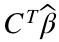, are derived from a matrix multiplication of the contrast matrix with the betas. This calculates the dot product between each contrast vector (independently) and the beta vector. The dot product is a measure of similarity between the vectors, scaled by the magnitude of each--so it tells us how strongly the beta vector "agrees" with each contrast. Another way to look at it is as a weighted average, where the contrast vector supplies the weights.
The contrast valuesreflect the magnitude of an effect of interest -- e.g., the magnitude of the difference in brain activity across two (or more) conditions. These values, for each participant, are what we'd typically take to a second-level (or group) analysis to make inferences about whether a particular brain area shows an effect in a population.
To do this for our simulated "face area" (for one participant), let's generate some simulated data and estimate the model parameters. We'll create a simulated signal that responds to only conditions A and C in our example. This corresponds to activation in response to non-inverted famous and non-famous faces. Note that we've named each contrast according to its semantic meaning in our experiment.
noise_ac = noise_arp(size(X, 1), [.7 .3]);
y = X(:, 1) + X(:, 3) + .2 * noise_ac; % simulated data
bhat = inv(X' * X) * X' * y; % Parameter estimates
yhat = X * bhat; % fitted values
create_figure('data and fits', 3, 1);
plot(y)
plot(yhat)
title('Data and fitted response')
% Plot the contrast values
% ---------------------------------------
subplot(3, 1, 2)
bar(C' * bhat)
contrast_names = {'Fam vs. nonfam' 'Upright vs. inverted' 'Interaction'};
set(gca, 'XTick', 1:3, 'XTickLabel', contrast_names, 'XTickLabelRotation', 45);
ylabel('Contrast value')
Each contrast is a linear combination of the regressors, so we can see what each looks like over time, too. Now let's apply the contrasts to our design matrix, X. This will show us what the contrast looks like over time. We'll plot it against the data:
Xc = X * C;
subplot(3, 1, 3)
plot(Xc)
title('Contrasts')
legend(contrast_names)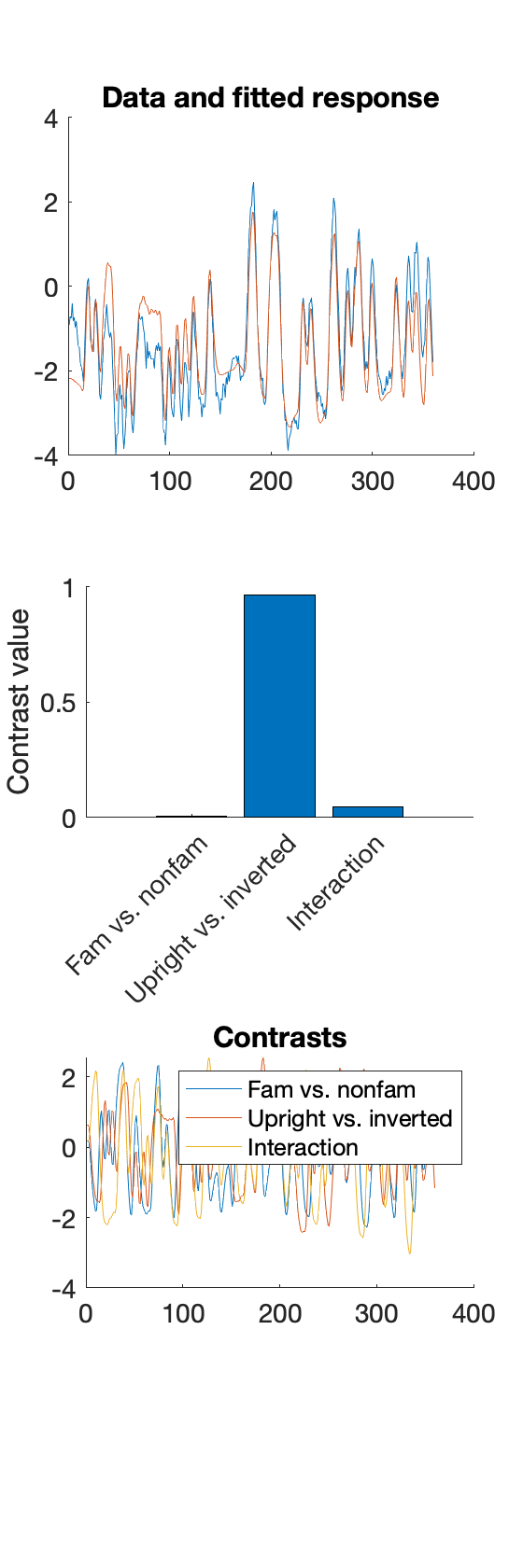Note: if you didn't adjust the design to include 4 event types, it won't work! You'll get an error saying something like: "Error using * Incorrect dimensions for matrix multiplication." This means you're trying to multiply two matrices that don't work together! For matrix multiplication, the number of columns of X must match the number of rows of C. Try:
whos X C
Then you can go back and fix the error by adjusting the design (or contrasts) appropriately.

1. What does Factor 1 represent, in terms of the semantic labels we assigned above? What contrast estimates it?
2. What about Factor 2? What contrast estimates it?
3. Which contrast has a high value (large effect magnitude)? Why?
4. Change the simulated data to show a large effect of Factor 1 (Famous vs. Non-) only.
5. If you change the contrast weights by, say, multiplying them by 2, what happens to the contrast values?

### Inference on contrasts

We estimated betas like so: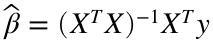, and contrasts like so:. We also estimated a t statistic for each beta (regression slope), which is a estimate divided by its standard error, or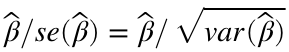. Furthermore,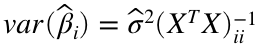. So we know how to perform inference on individual parameter estimates, but how do we tell if a contrast is statistically significant?
The t statistic for the contrast is calculated by applying the contrast weights to the betas and using the same formula. This turns out to be: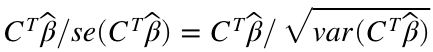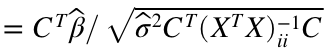For our example, this is:
sigma2 = (y - X * bhat)' * (y - X * bhat); % residual variance
con_t = C'*bhat ./ ( sigma2 .* diag(C'*inv(X'*X)*C) ).^.5;